# Adding and Subtracting Mixed Time

Print Rate 0 stars Common Core
Lesson size:
Message preview:
Someone you know has shared lesson with you:

To play this lesson, click on the link below:

Hope you have a good experience with this site and recommend to your friends too.

Login to rate activities and track progress.
Login to rate activities and track progress.

The easiest way to add and subtract mixed time is to set them up like an arithmetic problem and to borrow and carry numbers as needed.

The trick, though, is to remember that when you borrow or carry units, you have to convert them.

## Conversion rule:

Large unit to small unit: Multiply

Small unit to large unit: Divide

## Let's take a look at some examples:

### Example 1

What is the difference between 8 hours and 20 minutes?

### Solution:

First, we'll set it up as a subtraction problem. We need to line up the units.

Since they are separate units, we will not write them on top of each other:Now, we'll work from right to left just like a regular subtraction problem. But we cannot subtract

20 minutes from nothing, so we need to borrow. Notice, though, that we are borrowing 1 hour and bringing it over to the minutes column.

So, 1 hour is how many minutes?

It is 60 minutes.

So when we borrow 1 hour, we are borrowing 60 minutes.

We'll write that in: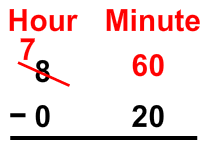Now we can subtract both columns: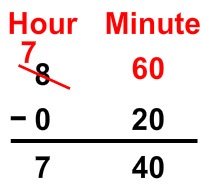### Example 2

It took 9 days for seedlings to appear after the seeds were planted. Then it took 3 weeks and 2 days for the plants to reach 5 inches. How long is this total?

### Solution:

We must first write this as an addition problem, lining up the weeks and the days;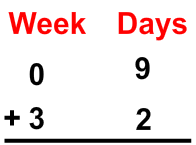Next, we add the days column. 9 + 2 = 11 days. But that is more than a week. Thus, we need to carry the remainder. There are 7 days in a week, so 11 days is 1 week plus 4 days. We'll carry the 1 week to the weeks column and put down 4 in the days column: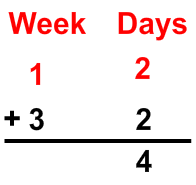And we'll finish by adding the weeks column: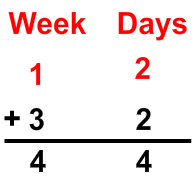### Example 3

Joe ran a mile in 7 minutes, 18 seconds. If he could take off 30 seconds from his time, what would his new time be?

### Solution:

First we must set up a subtraction problem. Remember to line up the minutes and seconds.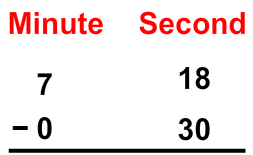Since we can't subtract 30 from 18, we must borrow from the 7 minutes. Remember that we are borrowing 1 minute, which is actually 60 seconds. Therefore, we'll need to add 60 seconds to 18.

18 + 60 = 78, so we'll change it to 78 seconds: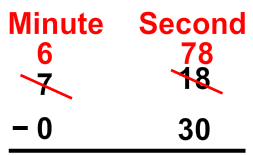Now we are ready to subtract each column: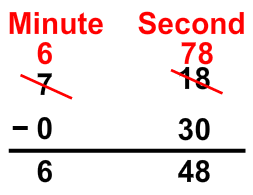## Adding and Subtracting Mixed Time

Time conversions:

• 60 seconds = 1 minute
• 60 minutes = 1 hour
• 24 hours = 1 day
• 7 days = 1 week
• 12 months = 1 year
• 52 weeks = 1 year
• 365 days = 1 year (366 for leap year)
• 10 years = 1 decade
• 100 years = 1 century
• 1000 years = 1 millennium

Conversion rule:

Large to small: Multiply

Small to large: Divide

## Similar Lessons

Become premium member to get unlimited access.